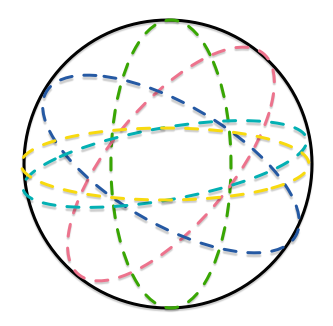Great CirclesGiven a sphere, a circle is called a great circle if it is the intersection of the sphere with a plane passing through its center. Five distinct great circles can dissect the sphere into $n$ regions.

Let $m$ and $M$ be the minimum and maximum values of $n$, respectively. Submit your answer as the concatenation of $M$ and $m$.

For example, if you think that $M=123$, $m=45$, then your answer is $12345$.

×